Latest Banking jobs   »   Quantitative Aptitude Quiz for IBPS 2020...

# Quantitative Aptitude Quiz for IBPS 2020 Mains Exams- 4th December

Directions (1-5): The following questions are accompanied by two statements (I) and (II). You have to determine which statements(s) is/are sufficient/necessary to answer the questions.
(a) Statement (I) alone is sufficient to answer the question but statement (II) alone is not sufficient to answer the question.
(b) Statement (II) alone is sufficient to answer the question but statement (I) alone is not sufficient to answer the question.
(c) Both the statements taken together are necessary to answer the question, but neither of the statements alone is sufficient to answer the question.
(d) Either statement (I) or statement (II) by itself is sufficient to answer the question.
(e) Statements (I) and (II) taken together are not sufficient to answer the question.

Q1. An article is marked 35% above its cost price, find the cost price of the article.
Statement I: Discount allowed on article is Rs.190 more than profit earned on the article.
Statement II: Ratio of selling price of the article to marked price of the article is 4 : 5.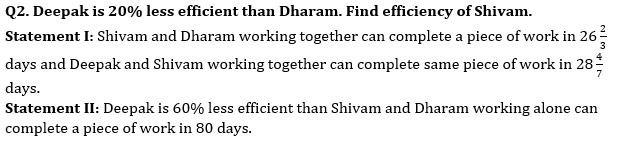Q3. Asif invested Rs.80000 across two schemes – A & B offering SI in the ratio 5 : 3 respectively. Find total interest earned by him.
Statement I: Rate of interest offered by scheme – B is twice of that of offered by scheme – A and period of investment in both schemes is 4 years.
Statement II: Interest received from scheme – B is Rs.6000 more than interest received from scheme – A.

Q4. Average of present age of Aman, Bhanu and Chaman is 34 years. Find present age of Chaman.
Statement I: Aman’s present age is 100% more than Chaman’s present age and ratio of Bhanu’s age 6 years hence to Chaman’s present age is 2 : 1.
Statement II: Sum of Aman’s and Bhanu’s present age is 78 years.

Q5. Ratio of length of train – A and train – B is 4 : 5. Find speed of train – A.
Statement I: Train – A can cross a 500m long platform in 28 seconds and train – A crosses train – B while running in same direction in 54 seconds.
Statement II: Train – B can cross a pole in 15 seconds.

Directions (6-10): The following questions are accompanied by two statements A and B. You have to determine which statements(s) is/are sufficient/necessary to answer the questions.
(a) Statement A alone is sufficient to answer the question but statement B alone is not sufficient to answer the questions.
(b) Statement B alone is sufficient to answer the question but statement A alone is not sufficient to answer the question.
(c) Both the statements taken together are necessary to answer the questions, but neither of the statements alone is sufficient to answer the question.
(d) Either statement A or statement B by itself is sufficient to answer the question.
(e) Statements A and B taken together are not sufficient to answer the question.

Q6. Find the overall profit or loss percentage of retailer if he sells two bats and four balls.
(A) Cost price of 3 bats is equal to selling price of 4 balls while profit percentage on selling one bat is 50%.
(B) On selling two bats retailer earn 50% profit while on selling four balls retailers’ loss 40% of his money.

Q7. A box contains 4 green ball, ‘x’ blue balls, 5 violet balls and ‘y’ yellow balls. Find the value of ‘x + y’.
(A) Probability of choosing two green ball is 1/20.
(B) Probability of choosing one blue ball or one violet ball is ½.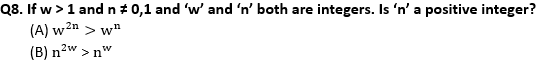Q9. Find the number of persons in a group if average weight of the group is 16?
(A) If one person left the group having weight 16 then average weight of the group is same as before.
(B) If two persons join the group having weight 16 and 28, then the average weight of group increases by 2.

Q10. In how many days ‘A’, ‘B’ and ‘C’ together can complete the work?
(A) A is 50% more efficient then B who is 100% more efficient than C while A and B together can complete the work in 12 days.
(B) ‘A’ and ‘B’, ‘B’ and ‘C’ and ‘C’ and ‘A’ together can complete the work in 12, 20 and 15 days respectively.

Directions (11-15): The following questions are accompanied by two statements (I) and (II). You have to determine which statements(s) is/are sufficient/necessary to answer the questions.
(a) Statement (I) alone is sufficient to answer the question but statement (II) alone is not sufficient to answer the questions.
(b) Statement (II) alone is sufficient to answer the question but statement (I) alone is not sufficient to answer the question.
(c) Both the statements taken together are necessary to answer the questions, but neither of the statements alone is sufficient to answer the question.
(d) Either statement (I) or statement (II) is sufficient to answer the question.
(e) Statements (I) and (II) together are not sufficient to answer the question.

Q11. Find Veer’s age four years hence from present?
(I) Ratio between Veer’s age and Rahul’s age, three years ago was 8 : 9, while Veer is two years younger than Rahul.
(II) Ratio between present age of Rahul and Veer is 21: 19, while five year hence average age of Rahul and Veer will be 25 years.

Q12. What will be profit share of Ankit out of total profit?
(I) Ankit Invest Rs. 1600 for 8 months and Satish joined him after third month with capital of Rs. 1200.
(II) Satish got Rs. 630 as profit share.

Q13. How many man and women working on this task?
(I) (x – 4) man work for first (x – 8) days, after that (x+2) woman complete the remaining work in (x – 8) days.
(II) Ratio between work did by men to work did by women is 2 : 3.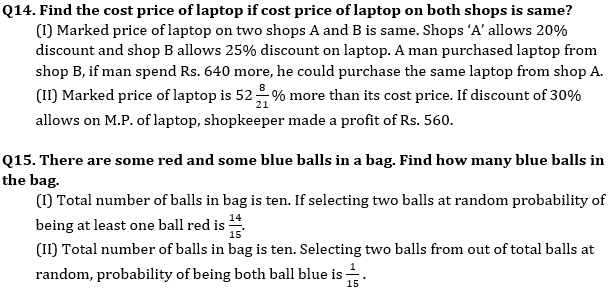Practice More Questions of Quantitative Aptitude for Competitive Exams:

Solutions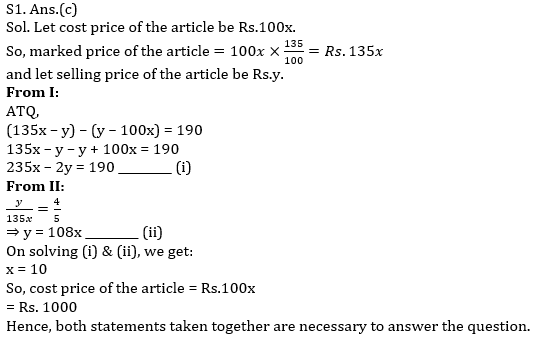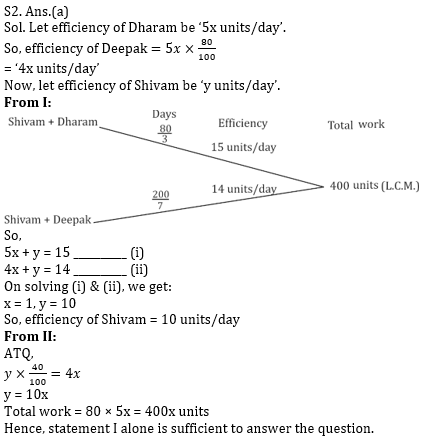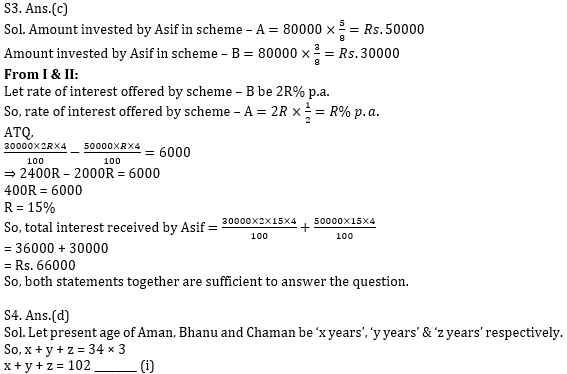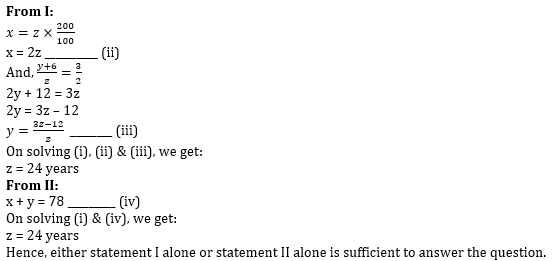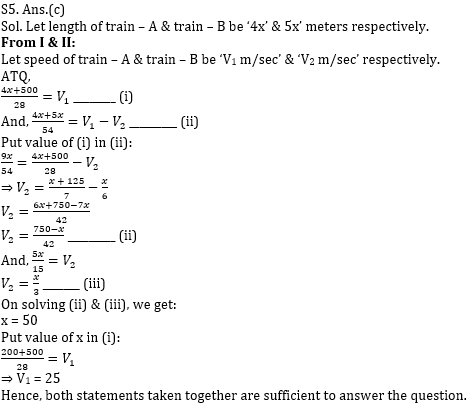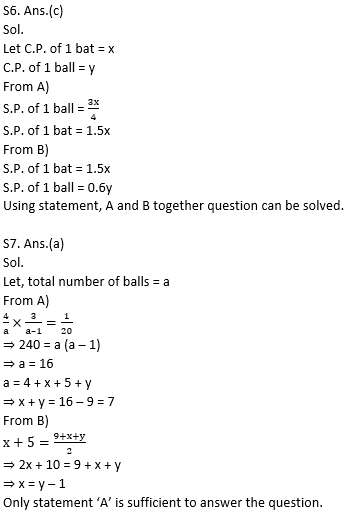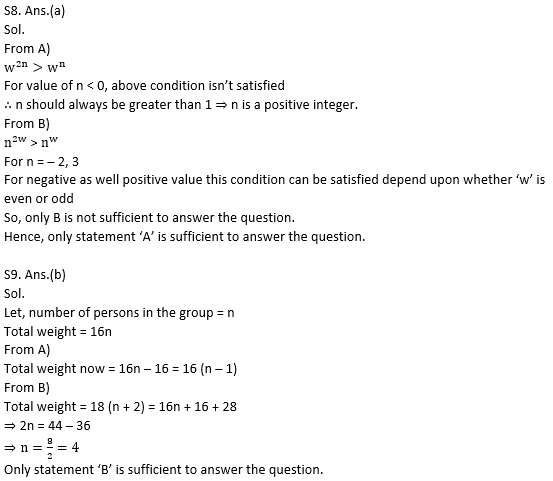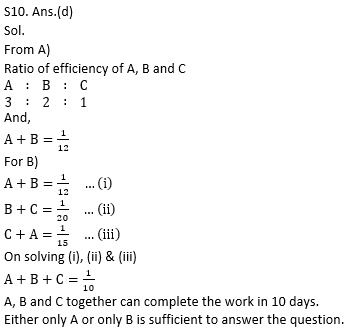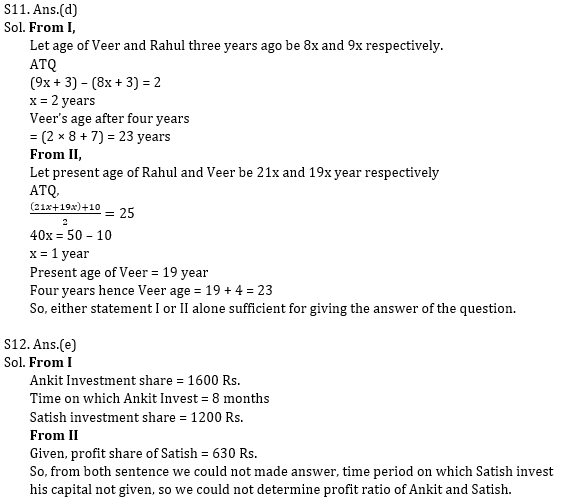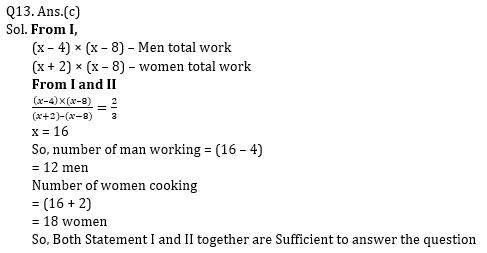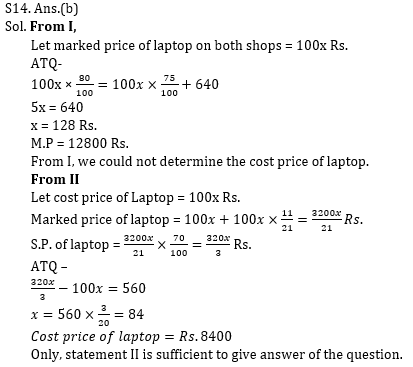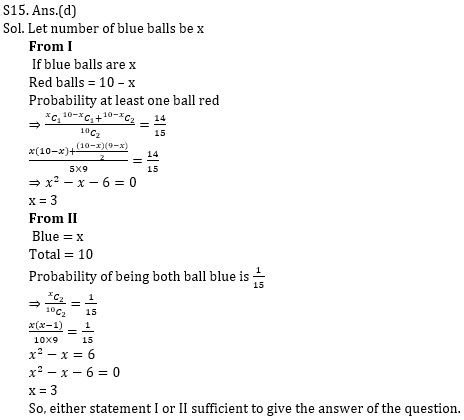Practice with Crash Course and Online Test Series for IBPS Clerk Prelims:×

Thank You, Your details have been submitted we will get back to you.Join India's largest learning destination

What You Will get ?

•Job Alerts
•Daily Quizzes
•Subject-Wise Quizzes
•Current Affairs
•Previous year question papers
•Doubt Solving session

ORJoin India's largest learning destination

What You Will get ?

•Job Alerts
•Daily Quizzes
•Subject-Wise Quizzes
•Current Affairs
•Previous year question papers
•Doubt Solving session

ORJoin India's largest learning destination

What You Will get ?

•Job Alerts
•Daily Quizzes
•Subject-Wise Quizzes
•Current Affairs
•Previous year question papers
•Doubt Solving session

Enter the email address associated with your account, and we'll email you an OTP to verify it's you.Join India's largest learning destination

What You Will get ?

•Job Alerts
•Daily Quizzes
•Subject-Wise Quizzes
•Current Affairs
•Previous year question papers
•Doubt Solving session

Enter OTP

Please enter the OTP sent to
/6

Did not recive OTP?

Resend in 60sJoin India's largest learning destination

What You Will get ?

•Job Alerts
•Daily Quizzes
•Subject-Wise Quizzes
•Current Affairs
•Previous year question papers
•Doubt Solving sessionJoin India's largest learning destination

What You Will get ?

•Job Alerts
•Daily Quizzes
•Subject-Wise Quizzes
•Current Affairs
•Previous year question papers
•Doubt Solving session

Almost there

+91Join India's largest learning destination

What You Will get ?

•Job Alerts
•Daily Quizzes
•Subject-Wise Quizzes
•Current Affairs
•Previous year question papers
•Doubt Solving session

Enter OTP

Please enter the OTP sent to Edit Number

Did not recive OTP?

Resend 60

By skipping this step you will not recieve any free content avalaible on adda247, also you will miss onto notification and job alerts

Are you sure you want to skip this step?

By skipping this step you will not recieve any free content avalaible on adda247, also you will miss onto notification and job alerts

Are you sure you want to skip this step?